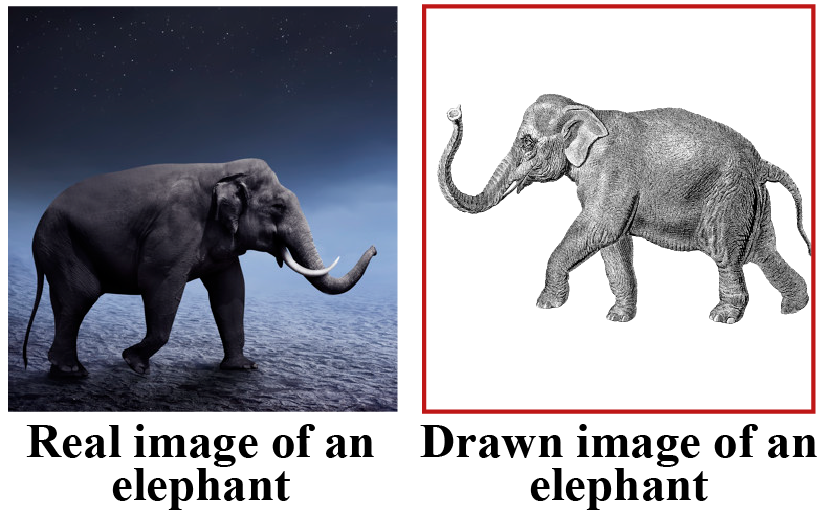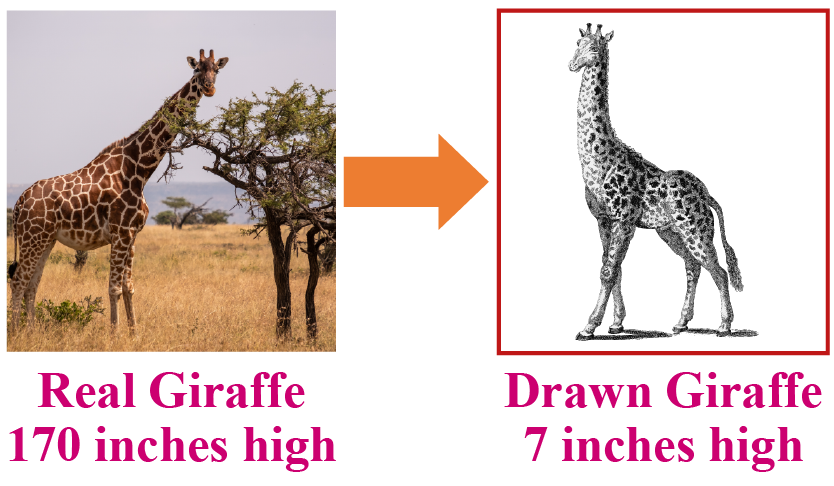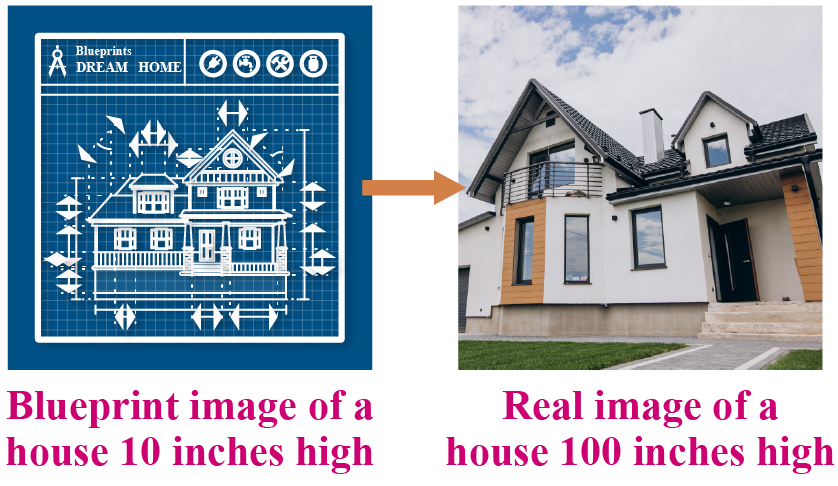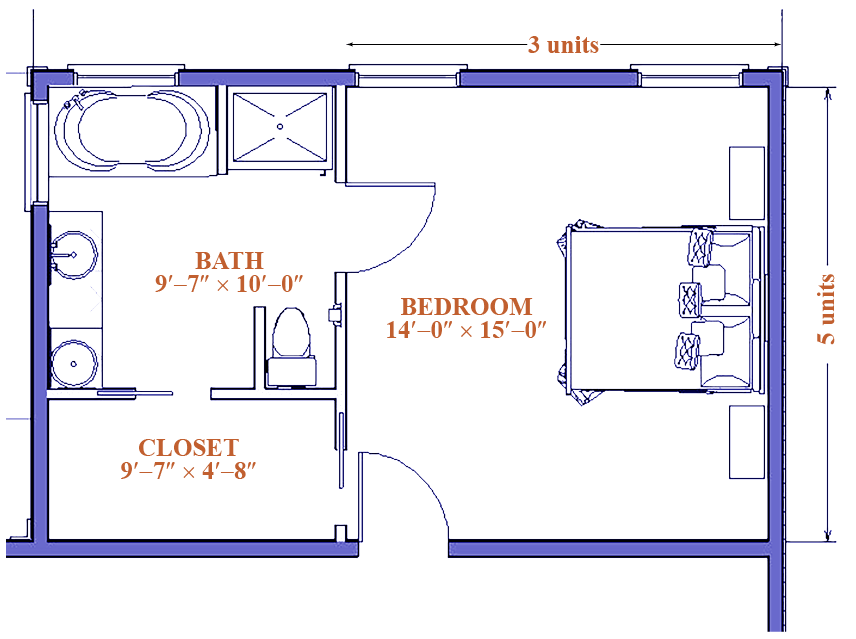# Scale

If you are asked to draw the image of an elephant by looking at a real elephant, will your dimensions on paper be the same as the huge creature? No, that's not possible. Therefore, when we draw the replica of the real-world images on paper, we use a ratio called scale.Scale ratio helps us in constructing buildings and representing the real world things on paper with comparatively smaller dimensions.

In this mini-lesson, we will explore the world scale drawings. We will walk through the definition of scale, how to use a scale for drawings, and how to interpret scale drawings

## What Is Meant By Scale?

### Scale Definition

Scale is defined as the ratio of the actual length of any object on the blueprint to the actual length of the same object in the real world.

When we draw a real-world thing on a piece of paper we use a scale to describe our measurements accurately. For example, Scale 1:10 means the size of 1 unit on paper would represent 10 units size in the real world.

For example,

If a giraffe with a height of 170 inches in the real world is represented as 7 inches on the drawing, the scale ratio for this case is $$7: 170$$.

The scale diagram for this case can be shown as:## How to Use a Scale For Drawings?

Let us say you want to construct a house. Before constructing a house in the real world, the first step is to draw a blueprint.

A blueprint is a drawing prepared with the help of a scale.

The following points describe how to use a scale for drawings:

• In the case of house construction, if a wall is 100 inches high in the real world and represented as 10 inches in the blueprint, the scale will be 10:100.
• The units and the dimensions used in the real world are greater as compared to the blueprint.

Observe the scale diagram for the same.Think Tank
1. Bosco is an artist. He decides to draw an elephant with a scale ratio of 3:20 inches on his graph paper. What is the actual height of the real world elephant in feet?## How to Interpret Scale Drawing?

Let us consider a situation in which Joe and Ross are interpreting a 100:1 scale blueprint of their new house. The dimensions of the master bedroom in the floor plan are 5 units by 3 units. What is the area of the master bedroom in the real world?

Step-1: For every 100 units on a real-world, the house represents 1 unit on the blueprint drawing.

$$100$$ units of the actual house $$= 1$$ unit on a blueprint drawingTherefore,

\begin{align}L &=100\times5=500\ units\\\ B &=100\times3=300\ units\\\text{Area} &=L\times B=500\times300\\\ &=1,50,000\ Sq.\ Units\end{align}

## Interactive Questions

Here are a few activities for you to practice.

## Let's Summarize

The mini-lesson targeted the fascinating concept of scale. The math journey around the scale starts with what a student already knows, and goes on to creatively crafting a fresh concept in the young minds. Done in a way that not only it is relatable and easy to grasp, but also will stay with them forever. Here lies the magic with Cuemath.

At Cuemath, our team of math experts is dedicated to making learning fun for our favorite readers, the students!

Through an interactive and engaging learning-teaching-learning approach, the teachers explore all angles of a topic.

Be it worksheets, online classes, doubt sessions, or any other form of relation, it’s the logical thinking and smart learning approach that we, at Cuemath, believe in.

## 1. What is a scale factor of 5?

The scale factor of five means the ratio of two corresponding figures is 1: 5, where the smaller one is 1 and the bigger one is five times greater.

## 2. What does a scale of 1:50 mean?

The scale of 1: 50 means that 1 unit on the blueprint will represent 50 units in the real world. For example, 1 ft of a dummy model is equal to 50 ft in the real world.

## 3. What does a scale of 1:30 mean?

The scale of 1: 30 means that 1 unit on the blueprint will represent 30 units in the real world.

More Important Topics
Numbers
Algebra
Geometry
Measurement
Money
Data
Trigonometry
Calculus